FREE shipping to on qualifying orders when you spend or more. All prices ex. VAT.

# Contact Angle Measurements on Uneven Surfaces

In this guide we will use the Ossila Contact Angle to measure a droplet on an uneven surface. For a more general overview of the theory behind contact angles and the measurement technique we use, please see our Contact Angle: Theory and Measurement Guide.

Before we begin this guide, we must understand the difference between a sloped surface and an uneven surface. Figure 1 shows three types of surface: flat, sloped and uneven.Figure 1: Droplets on three types of surface. Contact angle measurements are more difficult on an uneven surface.

A sloped surface is usually just any flat surface at an angle. This has a linear gradient and can be flattened by adjusting the tilt of the sample stage, or rotating the video or image in the Contact Angle software.

Conversely, an uneven surface is a surface with a non-linear gradient which cannot be flattened by adjusting its angle. A non-linear gradient means that a single linear baseline cannot be used for the entire width of a droplet. This is shown by the red line in Figure 1.

This guide will help you overcome the difficulties of using an uneven surface in your contact angle measurements. We assume that you have already recorded a video of a droplet, and are currently in the process of analysing it.

### Preparing for Analysis

Open the software and click the ‘Analyse’ tab in the top left of the window.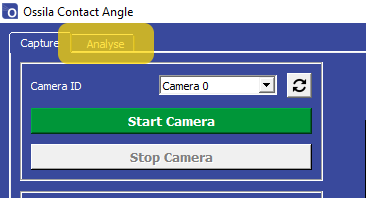Figure 2: The Analyse tab in the Ossila Contact Angle Software.

Load the video to be analysed using the ‘Load Video’ button. The video will appear in the central display. Navigate through the video frames until you find a frame that you would like to analyse. Figure 3 shows a droplet on a piece of cardboard that has variations in surface flatness.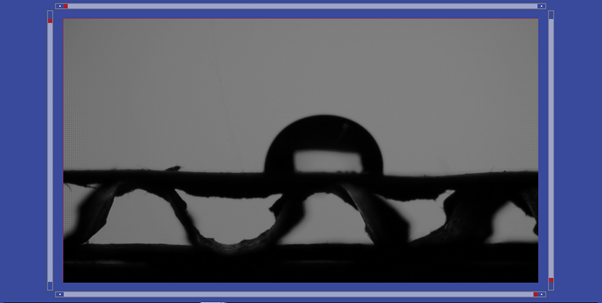Figure 3: Navigate to a frame with a droplet for analysis.

### Setting a Region of Interest

Move the region of interest (ROI) sliders until they surround the droplet as shown in Figure 4. The bottom horizontal line represents the baseline of measurement, which is the basis of the contact angle measurement. The left, right and top lines determine the part of the image that will be used in the measurement.

### Baseline Positioning

The contact angle measurement is performed at the point where the droplet edge meets the baseline. A magnified version of this region is displayed beneath the main viewer, as shown by the green boxes in Figure 5. A black and white threshold is applied to the magnified image, which allows us to see the droplet edge more clearly.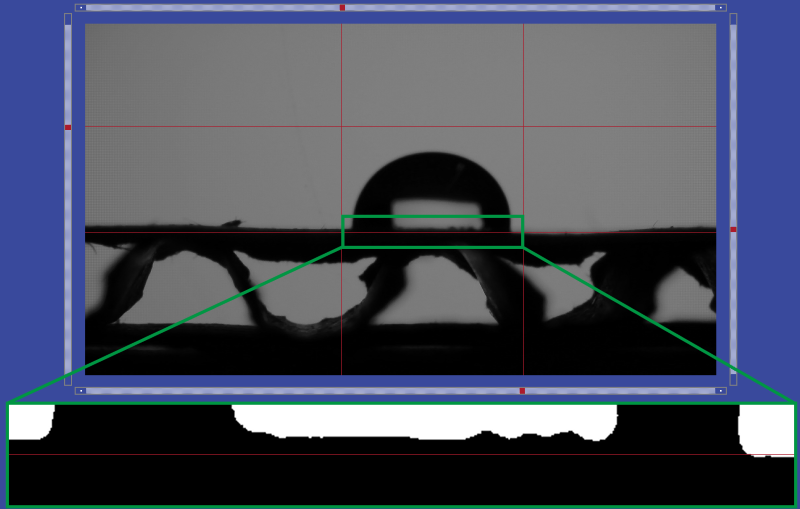Figure 5: The area above and below the baseline is displayed below the main viewer. This helps the user to position the baseline.

The baseline in Figure 5 intersects the right edge of the droplet at the point where it meets the substrate. On the left edge however, the baseline position is below the top of the substrate.

If the substrate was completely flat, then this difference could be due to the tilt of the stage, or the angle of the camera relative to the substrate. In that case, we would rotate the video so that the two contact points were level before performing the measurement.

In Figure 5 the flatness of the substrate varies beneath the droplet, so it is not possible to simply level the video or baseline. This variation is highlighted in Figure 6. Most of the droplet volume is on a flat area, but the right edge of the droplet is on an area that is sloped downwards relative to the left edge.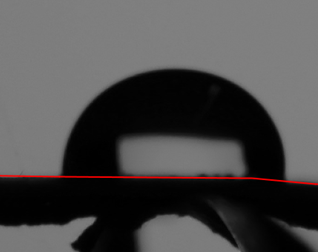Figure 6: A line highlighting the uneven surface of the substrate beneath the droplet.

One solution to this is to set the baselines for the two edges independently. We can perform the measurement on the left edge (Figure 7), then change the baseline position and perform the measurement on the right edge (Figure 8).Figure 7: Left edge baseline position.Figure 8: Right edge baseline position, which is lower than the left edge baseline position.

Another solution is to deposit a droplet on a flat area of the substrate. There is an area to the left of the droplet that would have been more optimal for deposition due to its flatness (Figure 9). If it is difficult to find a large and flat area, then reducing the volume of the droplet will reduce its diameter and make it easier to find a flat region of substrate in which it will fit.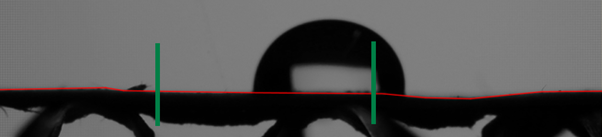Figure 9: A flat area of the substrate provides more accurate measurements.

### Improving the Polynomial Fit

The contact angle measurement involves fitting a polynomial to the edge of the droplet, as described in our Contact Angle Theory and Measurement Guide. The two most important ROI lines for this fitting are the bottom line (the baseline) and the top line. The bottom line defines where the contact angle measurement will be performed. The top line defined the part of the droplet edge that will be used for fitting the polynomial. Figure 10 shows what happens when we don’t choose our ROI carefully. The polynomial fit (red) is poor and the calculated angle is quite wrong.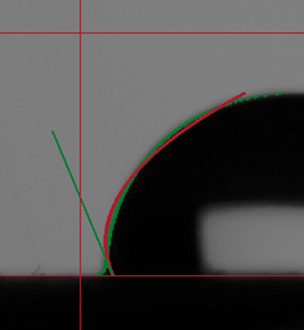Figure 10: Contact angle measurement with poor region of interest positioning.

With larger contact angles (angles above ~ 60°), you can get a much better fit if the top line is lowered to half way up the droplet, as shown by the red line in Figure 11. The polynomial fits much better to the edge data.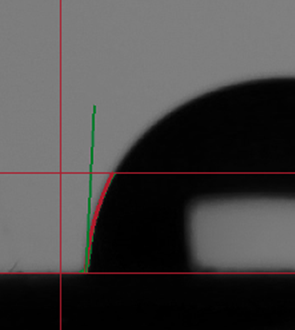Figure 11: Contact angle measurement using the bottom half of the droplet edge.

This is a much better fit, but we can still improve the accuracy of the measurement by looking at the polynomial plot in the bottom left of the Analyse tab (Figure 12). The plot shows the edge of the droplet in green and the polynomial fit in red. The right side of Figure 12 shows the intersection of the baseline with the droplet. We can see that there is a small meniscus formed between the droplet and the surface. This can happen if the droplet moves from its original position, or begins to evaporate and shrink after deposition. The meniscus reduces the accuracy of the polynomial fit, as the shape of the droplet edge with a meniscus is not well suited to a polynomial.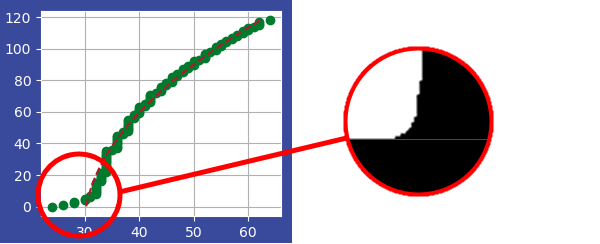Figure 12: Polynomial fit of the droplet shown in Figure 10. The droplet meniscus reduces the accuracy of the polynomial fit technique.

If we raise the baseline slightly, as shown in Figure 13, we can see that the polynomial fit matches the edge much better.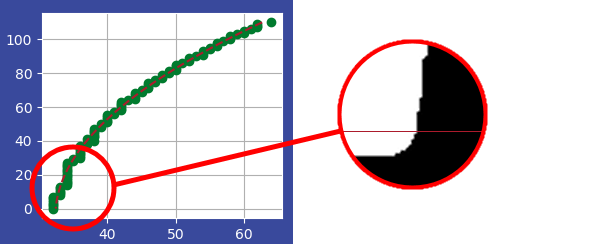Figure 13: Polynomial fit with no meniscus. The polynomial fit is much better when the baseline is raised slightly.

Using the ROI positioning method from Figure 13 and the separate-side technique shown earlier in this tutorial, we can analyse the left and right contact angles of the droplet. These are shown in the plot in Figure 14. The higher contact angle of the right side of the droplet is due to the slight slope which we saw in Figure 6.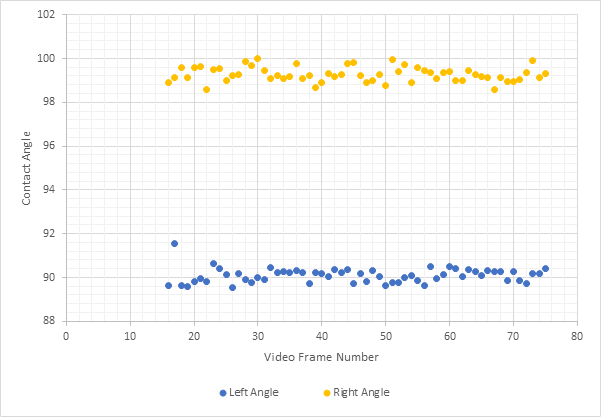Figure 13: Contact angles for the left and right of a droplet on an uneven surface.

### Conclusion

We successfully measured the contact angles of a droplet on an uneven surface by careful positioning of the region of interest in the Ossila Contact Angle software.